# Chapter 4 Estimation of solar radiation

Incident daily solar radiation is not interpolated, but estimated from topography and measurements of temperature, humidity and precipitation.

## 4.1 Solar declination and solar constant

The declination of the sun $$\delta$$ is the angle between the rays of the sun and the plan of the Earth’s equator. Solar declination varies with years and seasons. However, the Earth’s axial tilt changes slowly over thousands of years but it is nearly constant for shorter periods, so the change in solar declination during one year is nearly the same as during the next year. Solar constant ($$I_0$$) is normally given a nominal value of 1.361 $$kW \cdot m^{-2}$$ but in fact it also varies through the year and over years. Both can be calculated from Julian day ($$J$$), the number of days number of days since January 1, 4713 BCE at noon UTC. from Julian day. In meteoland, julian days, solar declination and solar constant are calculated using an adaptation of the code as in package insol by J.G. Corripio, which is based on and .

The following figures show the variation of solar declination and the value of solar constant over a year (see functions radiation_solarDeclination() and radiation_solarConstant()):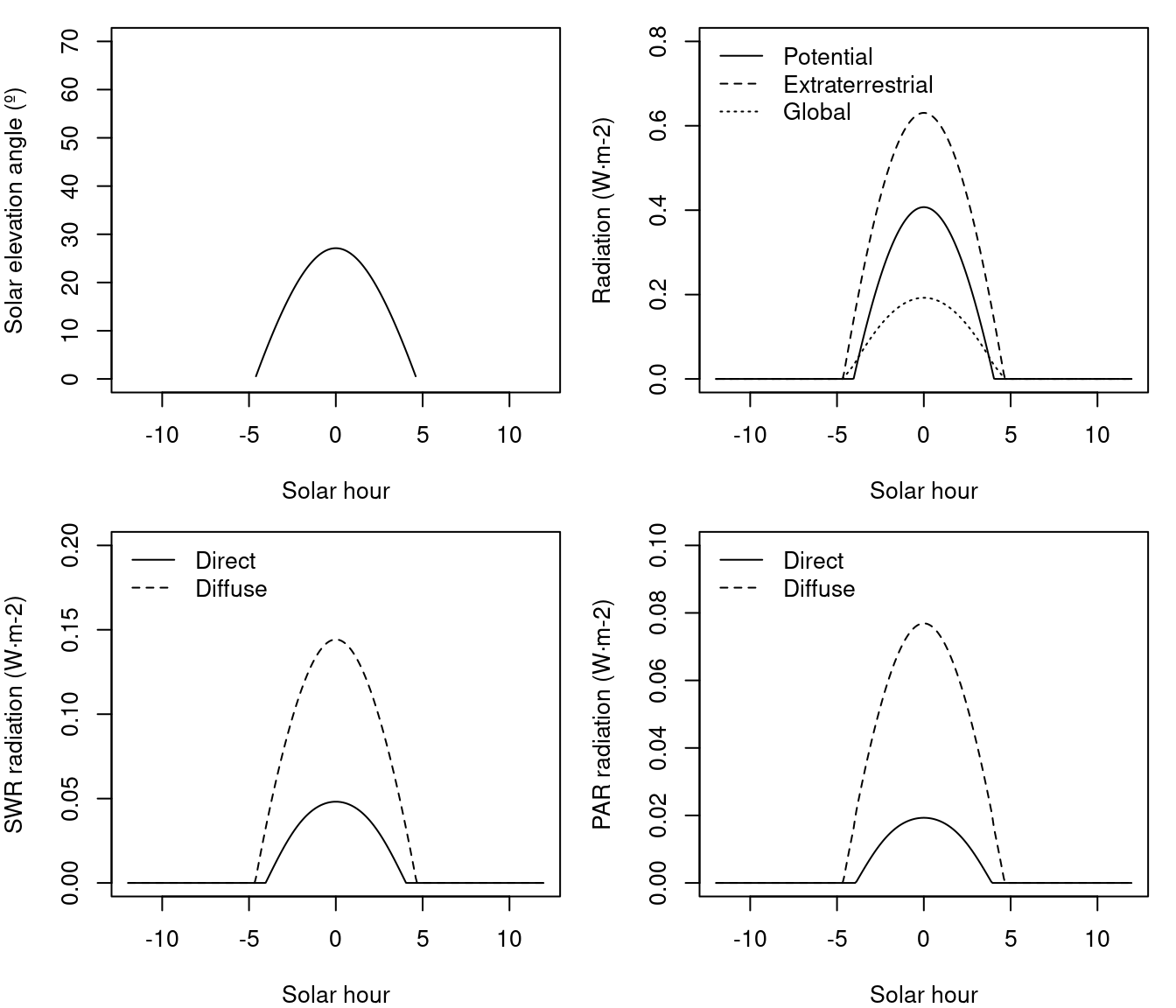## 4.2 Day length

Calculation of sunrise and sunset on a horizontal surface is rather straightforward. The hour angles of sunrise and sunset ($$sr$$ and $$ss$$, both in radians) for a horizontal surface of latitude $$\phi$$ on a day with declination $$\delta$$ (both expressed in radians) are: $\begin{eqnarray} sr &=& T_1 = \cos^{-1}\left(\max(\min(-\tan(\phi) \cdot \tan(\delta),1),-1)\right) \\ ss &=& T_0 = - T_1 \end{eqnarray}$ Knowing that each hour corresponds to 15 degrees of rotation, hour angles can be transformed to solar hours. The following figures show the seasonal variation of sunrise and sunset hours, as well as day length, for horizontal surfaces in three latitudes (40North, equator and 40South) (see functions radiation_sunRiseSet() and radiation_daylength()}):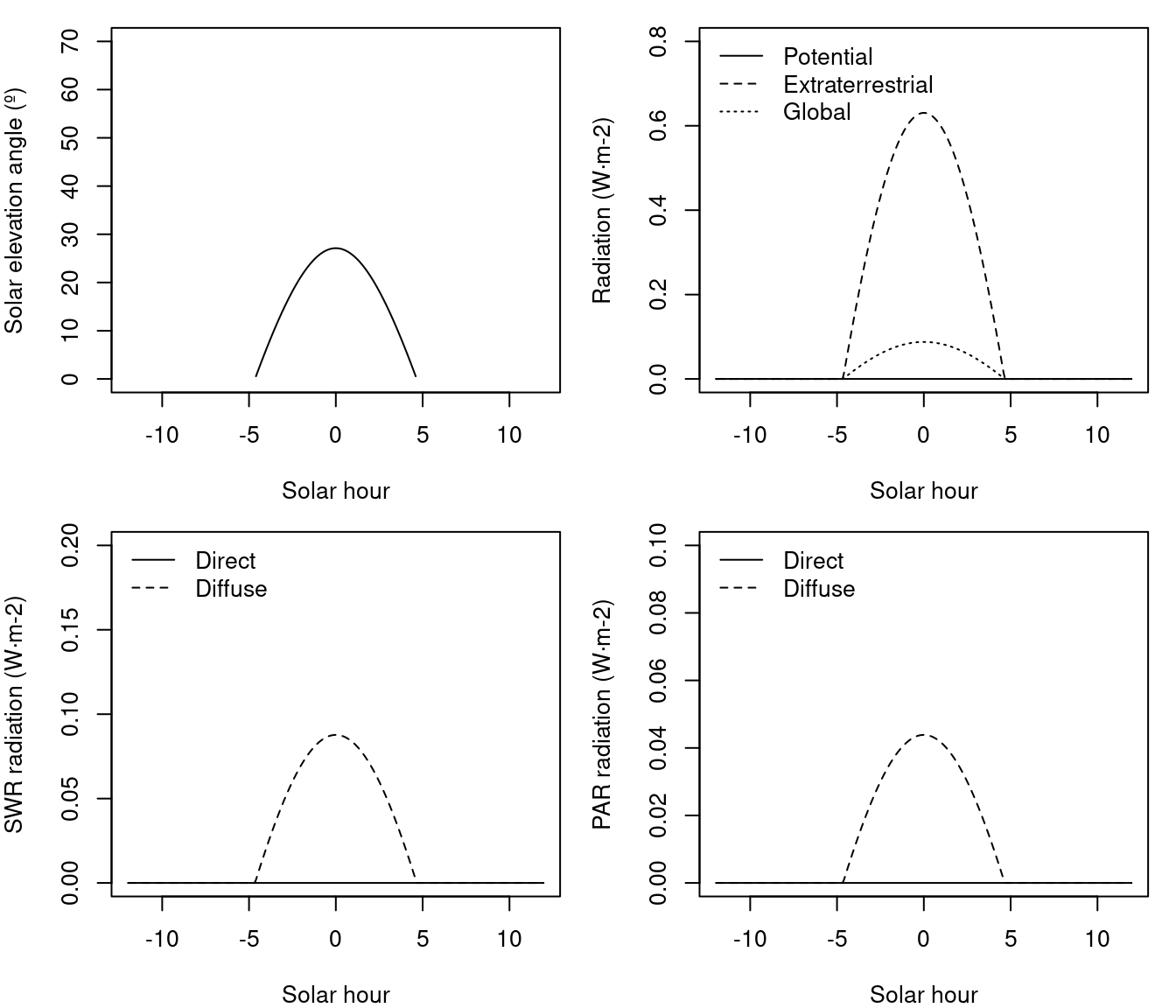For inclinated slopes, the calculation of day length is based on the concept of equivalent slopes, which are places on earth where the slope of earth’s surface is equal to the slope of interest. The calculations start with the determination of the latitude $$L_1$$ of the equivalent slope: $\begin{eqnarray} L_1 &=& \sin^{-1}\left(\cos(Z_x)\cdot \sin(\phi)+\sin(Z_x) \cdot \cos(\phi) \cdot \cos(A)\right) \\ D &=& \cos(Z_x) \cdot \cos(\phi)-\sin(Z_x) \cdot \sin(\phi) \cdot \cos(A) \end{eqnarray}$ where $$\phi$$ is the latitude, $$A$$ is the azimuth of the slope (aspect) and $$Z_x$$ is the zenith angle of the vector normal to the slope (equal to the slope angle). Then $$L_2$$ is defined depending on the value of $$D$$. If $$D < 0$$ then: $\begin{equation} L_2 = \tan^{-1}\left(\frac{\sin(Z_x) \cdot \sin(A)}{D}\right)+\pi \end{equation}$ Otherwise, $$L_2$$ is calculated as: $\begin{equation} L_2 = \tan^{-1}\left(\frac{\sin(Z_x) \cdot \sin(A)}{D}\right) \end{equation}$ Once $$L_1$$ and $$L_2$$ are available, we can calculate solar hours on equivalent slopes: $\begin{eqnarray} T_7 &=& \cos^{-1}\left(\max(\min(-\tan(L_1) \cdot \tan(\delta), 1),-1)\right)-L2 \\ T_6 &=& - \cos^{-1}\left(\max(\min(-\tan(L_1) \cdot \tan(\delta), 1),-1)\right) -L2 \end{eqnarray}$ Being $$T_6$$ and $$T_7$$ the hour angle of sunrise and sunset on equivalent slopes, respectively. and the hour angles of sunrise ($$sr$$) and sunset ($$ss$$) on the slope (both in radians) are found comparing the hour angles on equivalent surfaces with the hour angles on the horizontal surface: $\begin{eqnarray} sr &=& \max(T_0,T_6) \\ ss &=& \min(T_1,T_7) \end{eqnarray}$

The following three figures show the seasonal variation of sunrise and sunset hours, as well as day length, for slopes of 30 inclination, facing to the four cardinal points. Curves for flat surfaces are shown for comparison. If the slopes are at latitude 40North: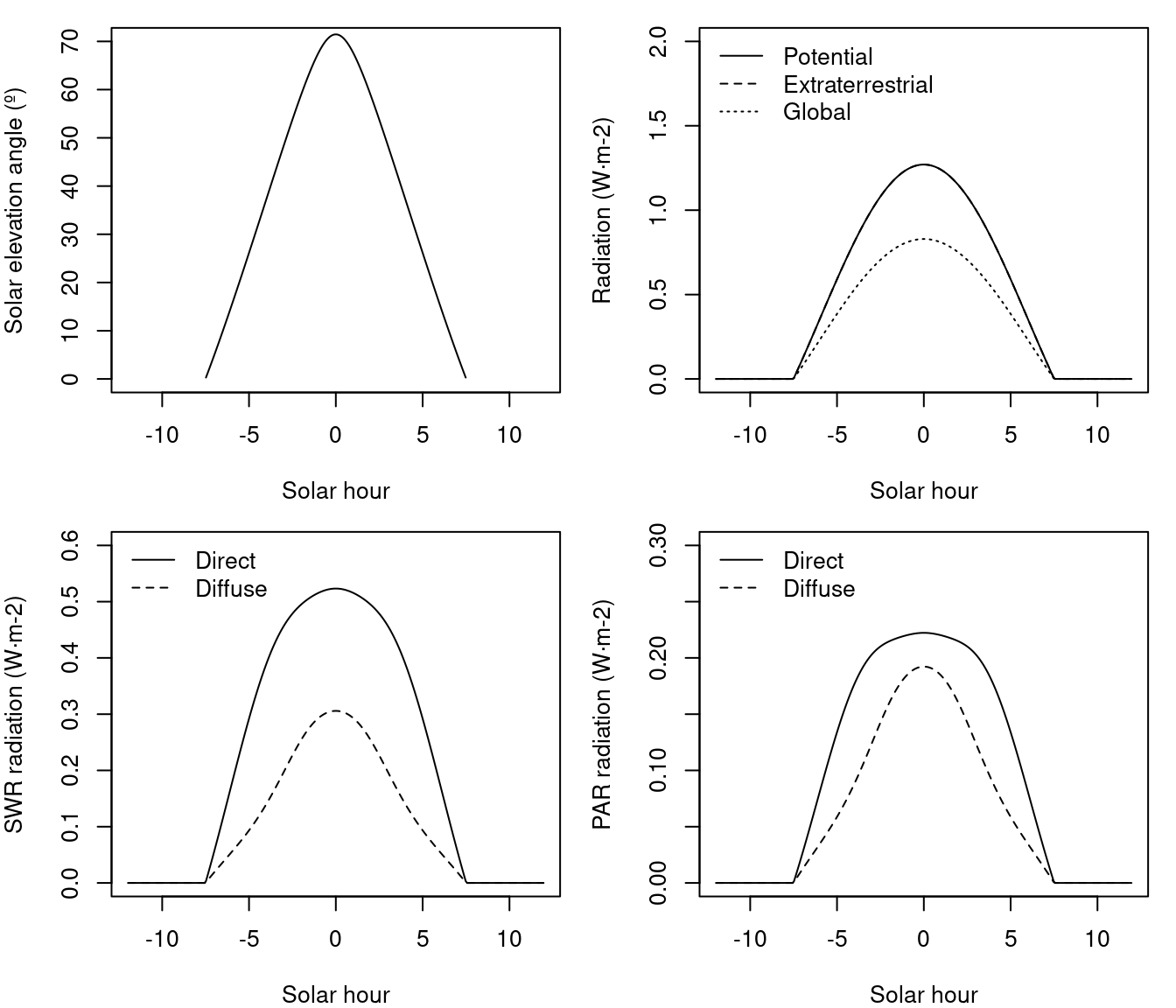whereas if they are at Equator (i.e. $$\phi = 0$$):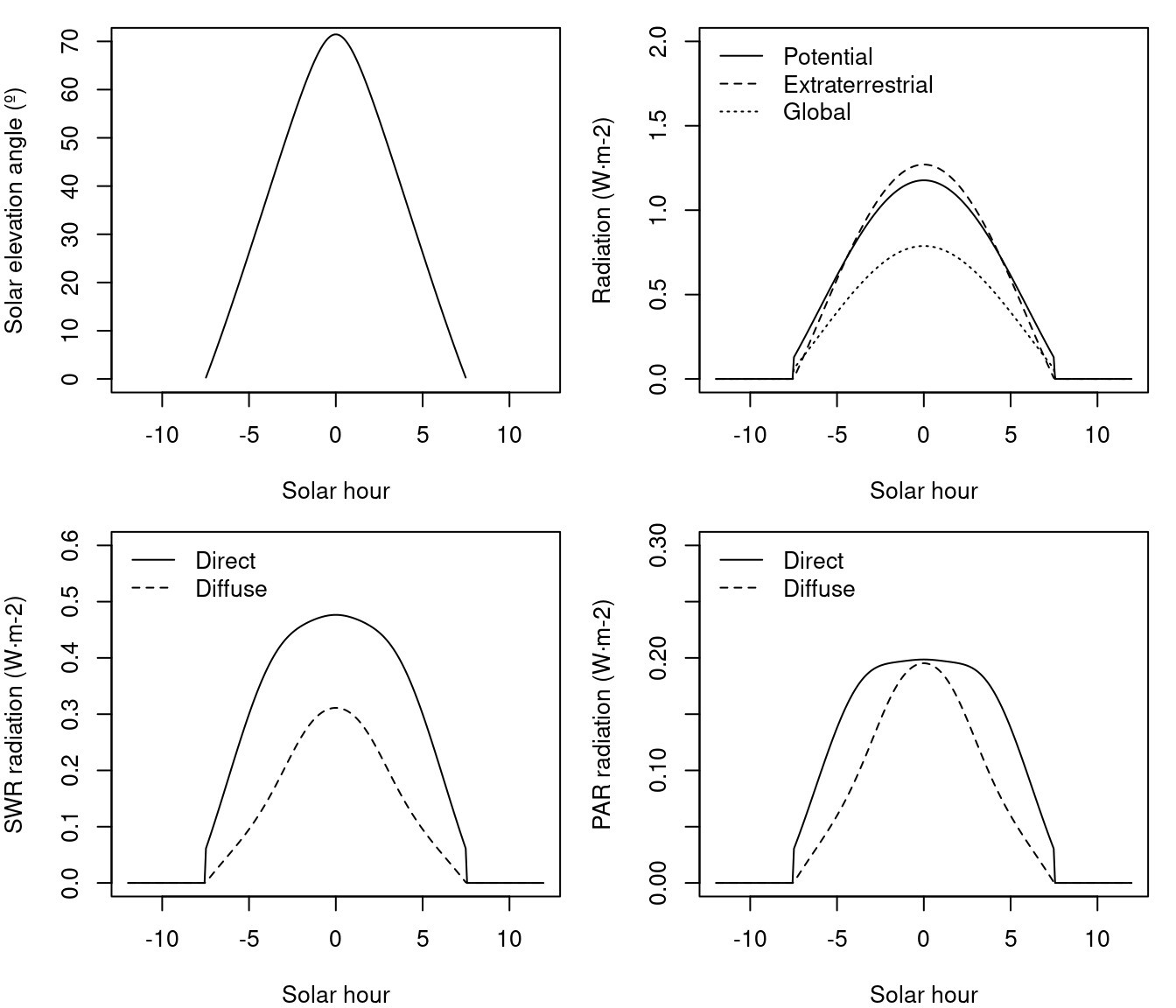and if they are at latitude 40South: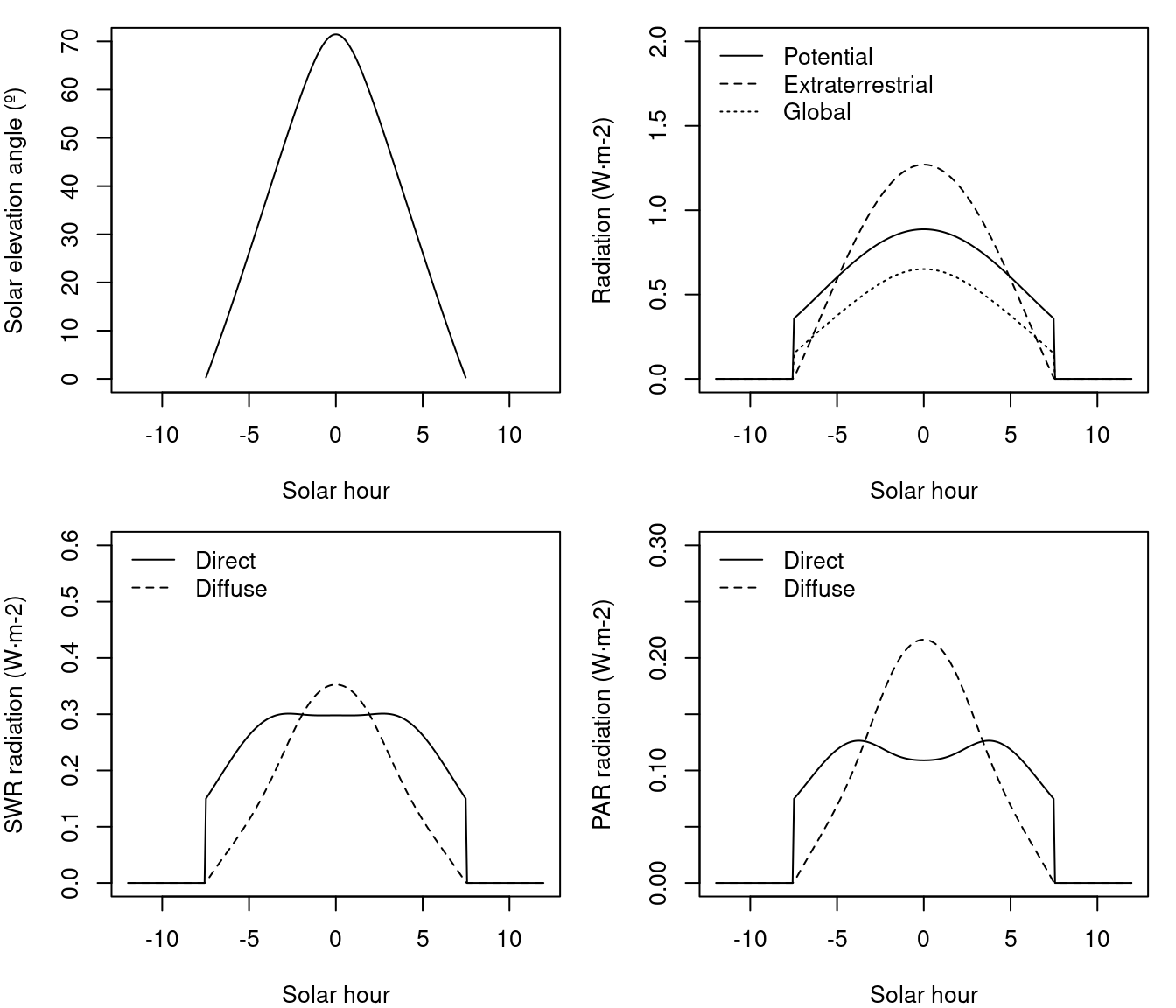Potential solar radiation is the radiation that a surface on earth would receive if atmosphere was not present (i.e. without the effects of cloud reflection, scattering, …). In meteoland, potential solar radiation is estimated from solar declination, latitude, aspect and slope according to . Daily potential radiation ($$R_{pot}$$, in $$MJ \cdot m^{-2}$$) is calculated by integrating instantaneous potential radiation $$R_{pot,s}$$ (in $$kW \cdot m^{-2}$$) over the day between sunrise ($$sr$$) and sunset ($$ss$$), using 10 min (i.e. 600 sec) intervals: $\begin{equation} R_{pot} = \frac{1}{1000}\cdot \sum_{s = sr}^{ss}{600 \cdot R_{pot,s}} \end{equation}$ In turn, instantaneous potential solar radiation $$R_{pot,s}$$ is calculated using: $\begin{eqnarray} R_{pot,s} &=& I_0 \cdot [(\sin{\phi}\cdot \cos{H})(-\cos{A}\cdot \sin{Z_x}) -\sin{H}\cdot (\sin{A}\cdot \sin{Z_x}) \nonumber \\ & & + [(\cos{\phi}\cdot \cos{H})\cdot \cos{Z_x}]\cdot \cos{\delta} \nonumber \\ & & + [\cos{\phi}\cdot (\cos{A}\cdot \sin{Z_x})+ \sin{\phi}\cdot \cos{Z_x}]\cdot \sin{\delta}] \end{eqnarray}$ where $$I_0$$ is the solar constant, $$\phi$$ is the latitude, $$H$$ is the hour angle measured from solar noon, positively towards the west, $$A$$ is the azimuth of the slope (aspect), $$Z_x$$ is the zenith angle of the vector normal to the slope (equal to the slope angle) and $$\delta$$ is the sun’s declination. Note that in the case of a flat surface the previous equation reduces to: $\begin{equation} R_{pot,s} = I_0 \cdot [\cos{\phi}\cdot \cos{H}\cdot \cos{\delta} + \sin{\phi}\cdot \sin{\delta}]= I_0 \cdot \sin{\beta} \end{equation}$ where $$\beta$$ is called the solar elevation angle.

The following figures illustrate seasonal variation of potential solar radiation for the horizontal inclinated surfaces presented above (see function radiation_potentialRadiation()):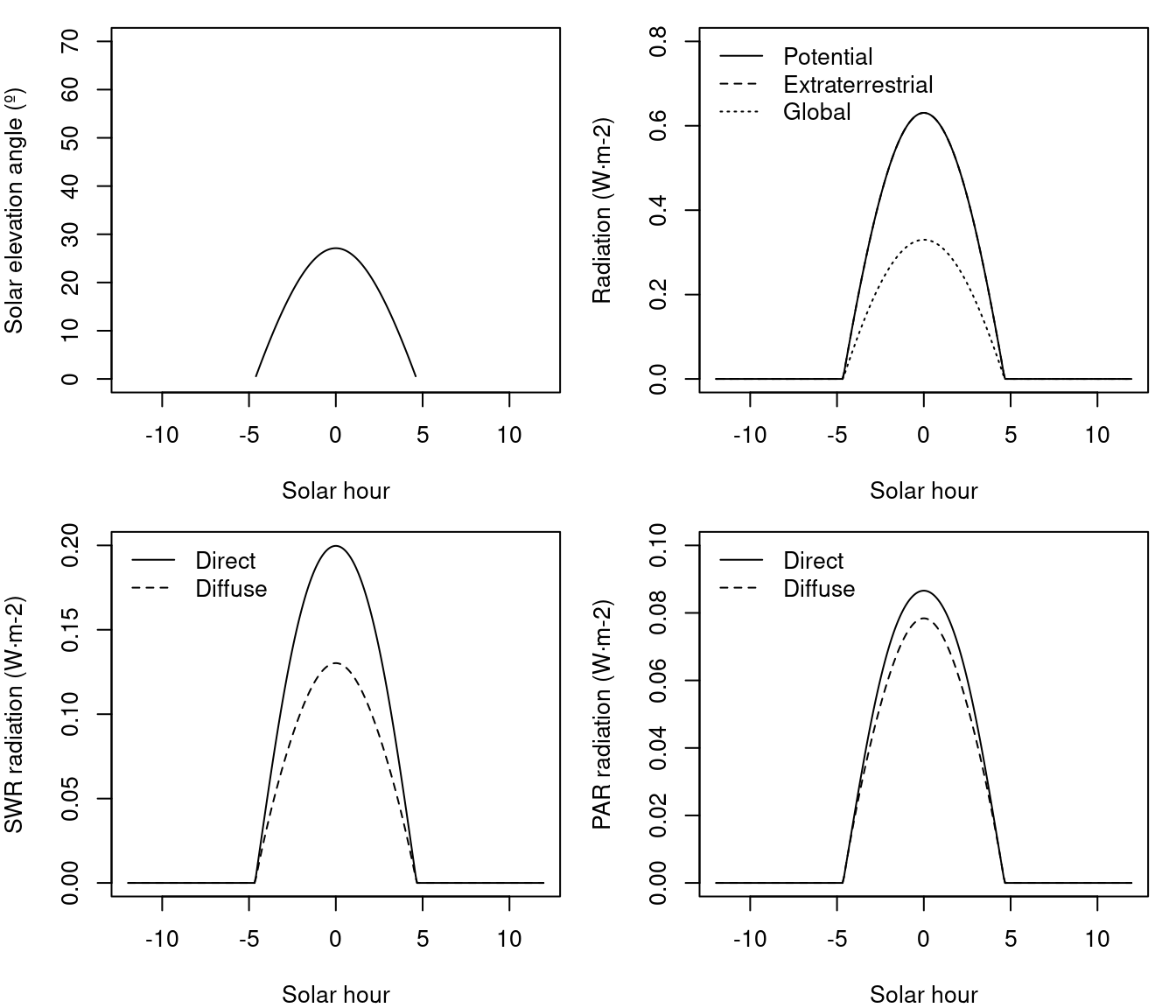Incident solar radiation is the amount of (direct) solar radiation reaching the surface after accounting for the atmosphere. Improving the method proposed in , calculate incident daily total solar radiation $$R_{g}$$ as: $\begin{equation} R_g = R_{pot} \cdot T_{t,max} \cdot T_{f,max} \end{equation}$ where $$T_{t,max}$$ is the maximum (cloud-free) daily total transmittance and $$T_{f,max}$$ is the proportion of $$T_{t,max}$$ realized on a given day (cloud correction). The maximum daily total transmittance $$T_{t,max}$$ is estimated as: $\begin{equation} T_{t,max} = \left[\frac{\sum_{s = sr}^{ss}{R_{pot,s} \cdot \tau^{(P_z/P_0)\cdot m_{\theta}}}}{\sum_{s = sr}^{ss}{R_{pot,s}}}\right] + (\alpha_{e_p} \cdot {e_p}) \end{equation}$ where $$\tau = 0.87$$ is the instantaneous transmittance at sea level, at nadir, for a dry atmosphere; $${e_p}$$ is the actual water vapor pressure (in kPa), estimated as explained before; $$\alpha_{e_p} = -6.1\cdot 10^{-2}$$kPa$$^{-1}$$ is a parameter describing the effect of vapour pressure on $$T_{t,max}$$; $$m_{\theta} = 1/\cos{\theta}$$ is the optical air mass at solar zenith angle $$\cos(\theta) = \sin{\phi}\cdot \sin{\delta}+\cos{\phi}\cdot \cos{\delta} \cdot \cos{H}$$; and $$P_z/P_0$$ is the ratio between air pressure at elevation $$z_p$$ and air pressure at the sea level, calculated as: $\begin{equation} (P_z/P_0) = (1.0 -2.2569\cdot 10^{-5}\cdot z_p)^{5.2553} \end{equation}$ In turn, $$T_{f,max}$$ was empirically related to $$\Delta T = T_{\max} - T_{\min}$$, the difference between maximum and minimum temperatures for the target point: $\begin{equation} T_{f,max} = 1.0 - 0.9\cdot e^{-B \cdot {\Delta T}^{C}} \end{equation}$ being $$C = 1.5$$ and $$B$$ calculated from: $\begin{equation} B = b_0 + b_1 \cdot e^{-b_2 \cdot {\hat{\Delta T}}} \end{equation}$ with $$b_0 = 0.031$$, $$b_1 = 0.201$$ and $$b_2 = 0.185$$. In this last equation, $$\hat{\Delta T}$$ is a 30-day moving average for the temperature range $$\Delta T$$. For computational reasons, we do not estimate $$\hat{\Delta T}$$ from the 30-day moving window average of predicted $$\Delta T$$ values, but from the interpolation of pre-calculated $$\hat{\Delta T}$$ values in weather stations. On wet days (i.e. if $$P_p > 0$$) the estimation of $$T_{f,max}$$ is multiplied by a factor of $$0.75$$ to account for clouds.

Although the calculation of incident solar radiation can be done independently of interpolation (see function radiation_solarRadiation()), it is automatically done in functions interpolationpoints() and interpolationgrid().

Potential and actual evapotranspiration calculations require estimating the energy actually absorved by evaporating surfaces. Daily net radiation $$R_n$$ (in $$MJ\cdot m^{-2}\cdot day^{-1}$$) is calculated using: $\begin{equation} R_n = R_s\cdot (1 - \alpha) - R_{nl} \end{equation}$ where $$R_s$$ is the input solar radiation (in $$MJ\cdot m^{-2}\cdot day^{-1}$$), $$\alpha = 0.08$$ accounts for surface albedo, and $$R_{nl}$$ is the net longwave radiation. Outgoing longwave radiation is the radiation emitted by earth. Following to obtain $$R_{nl}$$ one first calculates clear sky radiation $$R_{so}$$ using: $\begin{equation} R_{so} = (0.75 + \cdot 0.00002 \cdot z) \cdot R_{pot} \end{equation}$ where $$z$$ is elevation and $$R_{pot}$$ is potential radiation. $$R_{nl}$$ is then calculated using: $\begin{equation} R_{nl} = \sigma \cdot (0.34 - 0.14 \cdot \sqrt{e}) \cdot \frac{T_{\max}^4 + T_{\min}^4}{2} \cdot (1.35 \cdot \min(\frac{R_s}{R_{so}},1.0) - 0.35) \end{equation}$ where $$e$$ is the actual vapor pressure (kPa), $$T_{\max}$$ and $$T_{\min}$$ are the maximum and minimum temperatures (in Kelvin) and $$\sigma = 4.903\cdot 10^{-9} MJ \cdot K^{-4} \cdot m^{-2}$$ is the Stephan-Boltzmann constant.

The following figure shows an example of radiation balance for a whole year for a single site (see functions radiation_outgoingLongwaveRadiation() and radiation_netRadiation()`):### Bibliography

Danby, J. M. 1988. Fundamentals of Celestial Mechanics. 2nd ed. Richmond, VA: Willmann-Bell.
Garnier, B. J., and Atsumu Ohmura. 1968. A method of calculating the direct shortwave radiation income of slopes.” Journal of Applied Meteorology 7 (5): 796–800. https://doi.org/10.1175/1520-0450(1968)007<0796:AMOCTD>2.0.CO;2.
McMahon, T. A., M. C. Peel, L. Lowe, R. Srikanthan, and T. R. McVicar. 2013. Estimating actual, potential, reference crop and pan evaporation using standard meteorological data: a pragmatic synthesis.” Hydrology & Earth System Sciences 17: 1331–63. https://doi.org/10.5194/hess-17-1331-2013.
Reda, Ibrahim, and Afshin Andreas Nrel. 2008. Solar Position Algorithm for Solar Radiation Applications (Revised).” Nrel/Tp-560-34302, no. January: 1–56. https://doi.org/10.1016/j.solener.2003.12.003.
Spitters, C. J. T., H. A. J. M. Toussaint, and J. Goudriaan. 1986. Separating the diffuse and direct components of global radiation and its implications for modeling canopy photosynthesis. I. Components of incoming radiation.” Agricultural and Forest Meteorology 38: 231–42. https://doi.org/10.1016/0168-1923(86)90060-2.
Thornton, P. E., and S. W. Running. 1999. An improved algorithm for estimating incident daily solar radiation from measurements of temperature, humidity, and precipitation.” Agricultural and Forest Meteorology 93: 211–28. https://doi.org/10.1016/S0168-1923(98)00126-9.
Thornton, Peter E., Steven W. Running, and Michael a. White. 1997. Generating surfaces of daily meteorological variables over large regions of complex terrain.” Journal of Hydrology 190 (3-4): 214–51. https://doi.org/10.1016/S0022-1694(96)03128-9.